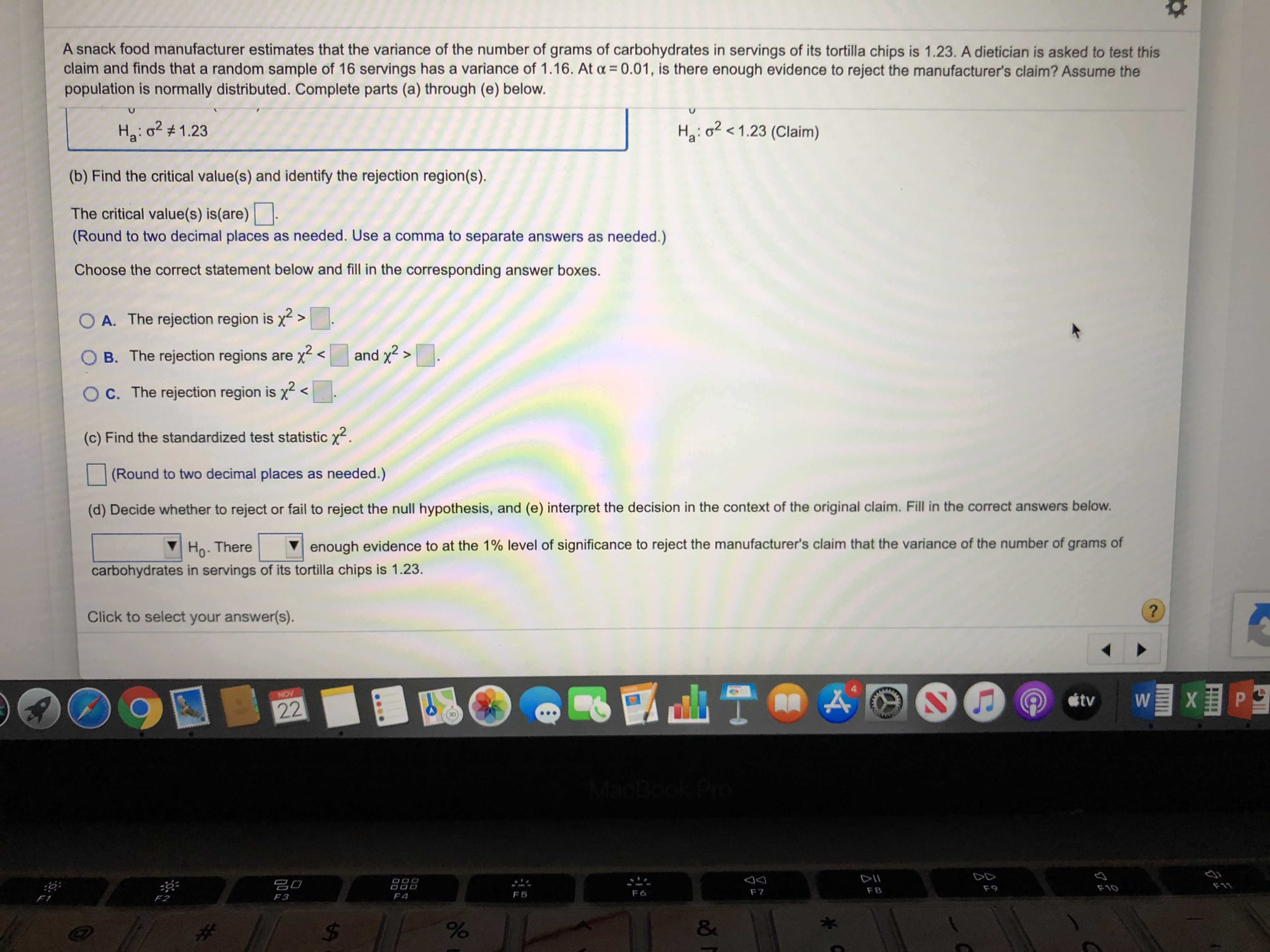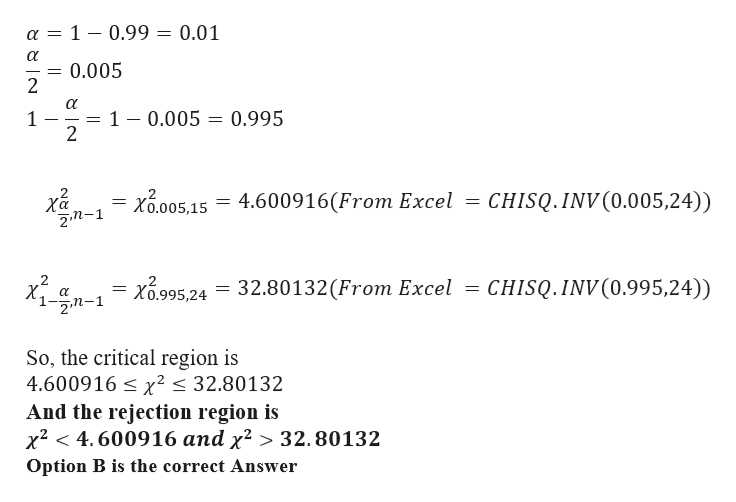# A snack food manufacturer estimates that the variance of the number of grams of carbohydrates in servings of its tortilla chips is 1.23. A dietician is asked to test thisclaim and finds that a random sample of 16 servings has a variance of 1.16. At a 0.01, is there enough evidence to reject the manufacturer's claim? Assume thepopulation is normally distributed. Complete parts (a) through (e) below.H2: o21.23 (Claim)Ha: o1.23(b) Find the critical value(s) and identify the rejection region(s)The critical value(s) is(are)(Round to two decimal places as needed. Use a comma to separate answers as needed.)Choose the correct statement below and fill in the corresponding answer boxes.OA. The rejection region is x2and x2>O B. The rejection regions are x <O C. The rejection region is x<(c) Find the standardized test statistic x(Round to two decimal places as needed.)(d) Decide whether to reject or fail to reject the null hypothesis, and (e) interpret the decision in the context of the original claim. Fill in the correct answers below.enough evidence to at the 1% level of significance to reject the manufacturer's claim that the variance of the number of grams ofHo. Therecarbohydrates in servings of its tortilla chips is 1.23.Click to select your answer(s).NOVtv22k ProDODIIO00O0D20F11F9F10F8F 7F6F5F4F3F2&#\$

Question
211 viewshelp_outlineImage TranscriptioncloseA snack food manufacturer estimates that the variance of the number of grams of carbohydrates in servings of its tortilla chips is 1.23. A dietician is asked to test this claim and finds that a random sample of 16 servings has a variance of 1.16. At a 0.01, is there enough evidence to reject the manufacturer's claim? Assume the population is normally distributed. Complete parts (a) through (e) below. H2: o21.23 (Claim) Ha: o1.23 (b) Find the critical value(s) and identify the rejection region(s) The critical value(s) is(are) (Round to two decimal places as needed. Use a comma to separate answers as needed.) Choose the correct statement below and fill in the corresponding answer boxes. OA. The rejection region is x2 and x2> O B. The rejection regions are x < O C. The rejection region is x < (c) Find the standardized test statistic x (Round to two decimal places as needed.) (d) Decide whether to reject or fail to reject the null hypothesis, and (e) interpret the decision in the context of the original claim. Fill in the correct answers below. enough evidence to at the 1% level of significance to reject the manufacturer's claim that the variance of the number of grams of Ho. There carbohydrates in servings of its tortilla chips is 1.23. Click to select your answer(s). NOV tv 22 k Pro DO DII O00 O0D 20 F11 F9 F10 F8 F 7 F6 F5 F4 F3 F2 & # \$ fullscreen
check_circle

Step 1

Given data
Population Variance σ2 = 1.23
sample Variance s2 = 1.16
no of samples (n)= 16
d.o.f = 15

a)

Formulation of Hypothesis

Null Hypothesis  H0: σ2 = 1.23 i.e. Variance of the number of grams of carbohydrates of tortilla chip is 1.23

Alternative Hypothesis ...help_outlineImage Transcriptionclosea = 1 0.99 = 0.01 0.005 2 1 0.005 0.995 1 2 2 CHISQ.INV(0.005,24)) 4.600916(From Excel χα 2,n-1 Χ.005,15 2 X 2m-1 CHISQ.INV (0.995,24)) x995,24 32.80132 (From Excel So, the critical region is 4.600916x2 32.80132 And the rejection region is x2 4.600916 and x2 > 32.80132 Option B is the correct Answer fullscreen

### Want to see the full answer?

See Solution

#### Want to see this answer and more?

Solutions are written by subject experts who are available 24/7. Questions are typically answered within 1 hour.*

See Solution
*Response times may vary by subject and question.
Tagged in

### Hypothesis Testing